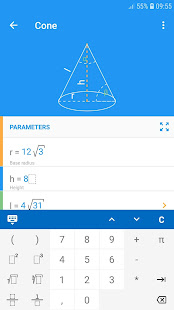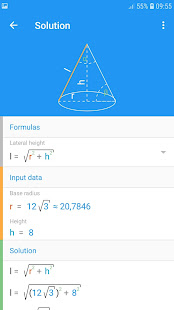# Math Studio v2.32 APK [Paid]

## Description

All in one. Step by step solution.
This app includes a lot of mathematics topics such as geometry, analytic geometry, equations and inequalities, quadratic function, linear function, linear system, circle equation, math sequences, algebra, vectors.
It also contains units calculator.

GEOMETRY
– Triangles: equilateral triangle, right triangle, isosceles triangle, 30-60-90
– Quadrilaterals: square, rectangle, rhombus, parallelogram, trapezoid, right trapezoid, isosceles trapezoid, kite
– Polygons: regular pentagon regular hexagon, regular octagon, regular dodecagon
– circle, ellipse, annulus and annulus sector
– Solids of revolution: sphere, cylinder, cone, truncated cone, barrel, spherical sector, spherical cap, spherical wedge, spherical lune, spherical segment, spherical zone
– Prisms: cube, square prism, cuboid, triangular prism, regular triangular prism, hexagonal prism, pentagonal prism
– Pyramids: regular tetrahedron, triangular pyramid, square pyramid, hexagonal pyramid
– Others: Pythagorean theorem, Thales’ theorem, trigonometry, law of sines, law of cosines

EQUATIONS AND INEQUALITIES
– First and second degree
– Linear equation
– Linear inequality
– Equations with parameter

ANALYTIC GEOMETRY
– Points and lines
– Intersection point
– Distance from point
– Length of the segment
– Parallel and Perpendicular line
– Perpendicular bisector
– Axial symmetry
– Central symmetry
– Translation by a vector
– Angle between lines

– standard form
– vertex form
– factored form
– discriminant of the quadratic function
– real roots (zeros)
– vertex of a parabola
– intersection of the Y-Axis
– monotonicity (increasing, decreasing)
– positive and negative values (inequalities)

LINEAR FUNCTION
– slope-intercept form
– standard form
– distance between two points
– midpoint of a line segment
– line segment bisector
– parallel line
– perpendicular line
– distance from a point to a line
– equation of the line passing through 2 points

LINEAR SYSTEM
Four methods for solving systems:
– Substitution method
– Elimination method
– Graph method
– Method of determinants

CIRCLE EQUATION
– standard form
– general form
– tangent line to the circle

MATH SEQUENCES
– properties of geometric progression: initial term, any mth term and nth term, ratio, sum of n terms, general formula
– properties of arithmetic progression: initial term, any mth term and nth term, difference, sum of n terms, general formula
– properties of geometric series: initial term, ratio, sum

ALGEBRA
– greatest common divisor (gcd)
– least common multiple (lcm)

VECTORS
– 2D and 3D
– Length of a vector
– Dot product
– Cross product

UNITS (calculator)
– Length, distance
– Mass
– Velocity
– Power
– Pressure
– Temperature
– Time
– Energy
– DataMath Studio v2.32 APK [Paid]

[toggle title=”Old Versions” state=”close”]

Math Studio v2.30 APK [Paid]

[/toggle]